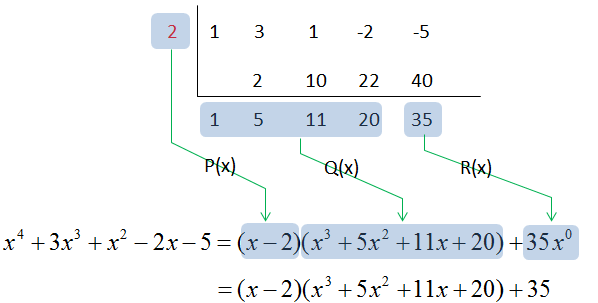High School Math-Advanced Function-Polynomial Function What is Polynomial Function ?   Polynomial Function is an expression of more than two algebraic terms, especially the sum of several terms that contain different powers of the same variable(s). it can be express in a generic form as shown below.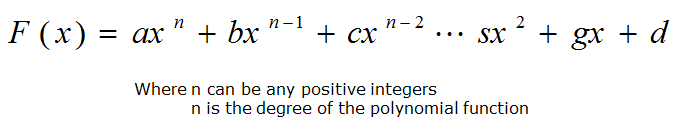How to Graph ?   You can graph any polynomial functions by both hands(harder method) and technology(easier method).   When graphing with hands, you need to know the x-intercepts(also known as roots), y-intercept, and end behaviours of the function. Some of the tips you can use for drawing by hands are When a polynomial function is in its standard form, you must factor the equation in order to find the roots You can use both synthetic division and long division to factor the function If a polynomial function is an even degree function with positive sign, the end behaviour is quadrant 2 to quadrant 1. However if the function has a negative sign, the end behaviour is quadrant 3 to quadrant 4 If a polynomial function is an odd degree function with positive sign, the end behaviour is quadrant 3 to quadrant 1. However if the function has a negative sign, the end behaviour is quadrant 2 to quadrant 4 End behaviours are ALWAYS from left to right < Quadrant on a Cartesian Coordinate >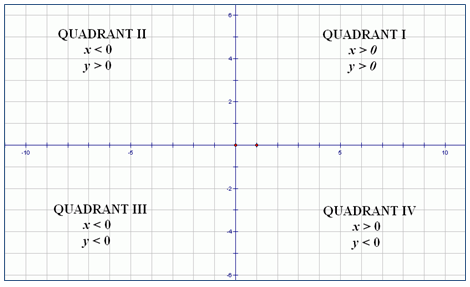Descartes rule of signs   This rule is used to identify the number of positive roots, negative roots, and/or imaginary Roots of a polynomial function. By the application of this rule, you can identify whether you found the correct roots or not.   If a polynomial function of f(x) has n sign changes, there are n positive roots If f(-x) has a sign changes, there are a negative roots Degree of function = total Number of positive and Negative root(s) and number of imaginary root(s)     Even and odd functions   Odd function is an odd-degree function with a point symmetry, proved by f(-x)=-f(x), x can be any real numbers   Even function is an even-degree function with a line symmetry, proved by f(x) = f(-x), x can be any real numbers     Polynomial Division   < Synthetic Division >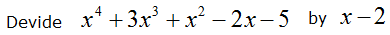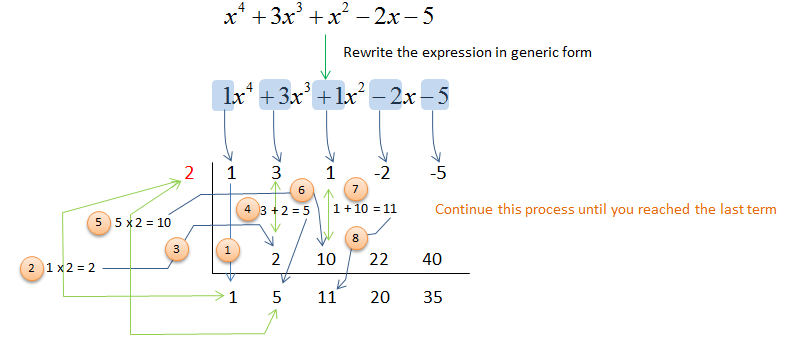Write the answer in P(x)Q(x)+R(x) format